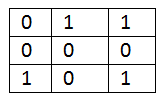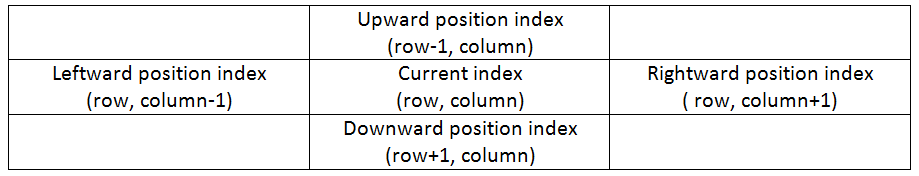# Exit Point in a Matrix

In this article, we are going to see a maze problem (exit point in a matrix) which came in the coding round of Samsung.
Submitted by Radib Kar, on December 10, 2018

Problem statement:

Given a matrix with 0's and 1's, one enters the matrix at cell (0, 0) in left to right direction. Whenever one encounters a 0 he retains in the same direction, if one encounters a 1 he has to change direction to the right of current direction and change that 1 value to 0. Write a programming out from which index he will leave the matrix at the end. (Indexing starts from 0).

Input: An mxn matrix with each element 0 or 1

Output: Exit cell index pair (i, j) where is the row no & j is the column no

Example & discussion

Let's consider a 3x3 matrix of each element 0/1.Given that:

• Starting position is (0, 0).
• Starting moving direction is left to right (rightwards).
• Whenever current element value is 0, it retains its direction.
• Whenever current element value is 1, it changes direction to right of current moving directions.
• Moving directions can be of four types
• Leftwards
• Rightwards
• Downwards
• Upwards
• Whenever current element value is 0, moving directions becomes like
• Leftwards → leftwards
• Rightwards → rightwards
• Upwards → upwards
• Downwards → downwards
• Whenever current element value is 1, moving directions becomes like
• Leftwards → upwards (changing to right direction)
• Rightwards → downwards (changing to right direction)
• Upwards → rightwards (changing to right direction)
• Downwards → leftwards (changing to right direction)
• Current element value: 1 → 0

So, let's solve the above example,

```    1st move:
Current element: 0
Current index: (0, 0) (starting index)
Moving direction: rightwards (starting moving direction)
Next index: (0, 1)
Next element: 1

2nd move:
Current element: 1->0
Current index: (0, 1) (starting index)
Moving direction:  downwards (changing direction)
Next index: (1, 1)
Next element: 0

3rd move:
Current element: 1->0
Current index: (0, 1) (starting index)
Moving direction:  downwards (changing direction)
Next index: (1, 1)
Next element: 0

4th move:
Current element: 0
Current index: (1, 1)
Moving direction:  downwards (no change in direction)
Next index: (2, 1)
Next element: 0

5th move:
Current element: 0
Current index: (2, 1)
Moving direction:  downwards (no change in direction)
Next index: out of the matrix
So, we are done & the exit point is (2, 1)
```

Algorithm:

1. We start from the starting index & a moving direction rightwards.
2. Check the current element value
3. If current element value == 0
• No change in moving direction
• Find next index value
Else
• Change in moving direction
• Find moving direction based on current direction
• Find next Index value
4. If next index value is out of boundary
• Set current index value as exit point index & print it. Return.
Else
• Set current index value to next index value (for next move)
• Set current element value to next element value (for next move)
• Repeat step 2-4

The major things in the program are:

1. To check whether we have moved out of the matrix (exit point reached)
2. How to find the changed direction for moving next

First one can be solved by keeping check for boundary indexes like:

```    If (row of index<0)
Out of matrix;
If (column of index<0)
Out of matrix;
If (row of index>=m) //m is row no of matrix
Out of matrix;
If (column of index>=n) //n is column no of matrix
Out of matrix;
```

Now addressing to the second one, change in moving direction, and the following table helps up to understand,All the directions are marked on basis of the current index.

```    For rightward move->(row, column+1)
For downward move ->(row+1, column)
For leftward move ->(row, column-1)
For upward move->(row-1, column)
```

So we can handle this using two direction arrays and keeping a counter

```    Row_direction= [0, 1, 0, -1];
Column_direction= [1, 0, -1, 0];
Counter=0;
```

So, initially moving direction is [0, 1], (rightwards) i.e., for every move

Next index= (current_index_row+0, current_index_column+1)

Until there is no change to counter direction, counter is unchanged.

In case there is a change,

Set counter= (counter+1)% 4;

(mod 4 is done to love back to initial direction after changing for 4 times, like rightward->downward->leftward->upward->rightward->…….so on)

## C++ implementation for Exit Point in a Matrix

```#include <bits/stdc++.h>
using namespace std;

int outofMatrix(int row,int column,int m,int n){
//all cases for index to be out of matrix
if(row<0)
return 1;

if(column<0)
return 1;

if(column>=n)
return 1;

if(row>=m)
return 1;

//else
return 0;
}

void exitInMatrix(int** a,int m,int n){
int row_direction={0,1,0,-1};
int column_direction={1,0,-1,0};

int counter=0;//starting moving direction index
//starting index && next index for if starting value 0
int next_column=1,next_row=0,current_column=0,current_row=0;
if(a==1){//when starting element ==1, direction becomes downwards
next_column=0;
next_row=1;
counter=1; //to indicate downward movement
}

while(!outofMatrix(next_row,next_column,m,n)){//until we haven't exited
//update current_column, current_row
current_column=next_column;
current_row=next_row;
if(a[current_row][current_column]==1){//if current element 1
a[current_row][current_column]=0;
counter=(counter+1)%4; //update counter value
next_column=current_column+column_direction[counter];
next_row=current_row+row_direction[counter];
}
else{//if current element 0
next_column=current_column+column_direction[counter];
next_row=current_row+row_direction[counter];
}
}
printf("%d %d\n",current_row,current_column);//print exit index
}

int main(){
int n,m;

scanf("%d %d",&m,&n);
int **a=(int**)malloc(sizeof(int*)*m);
for(int i=0;i<m;i++)
a[i]=(int*)malloc(sizeof(int)*n);

for(int i=0;i<m;i++){
for(int j=0;j<n;j++){
scanf("%d",&a[i][j]);
}
}

exitInMatrix(a,m,n);

return 0;
}
```

Output 1

```enter rwo & column no of matrix
3 3
enter elemnts, 0 or 1
0 1 1
0 0 0
1 0 1
exit point: (2 1)
```

Output 2

```enter rwo & column no of matrix
4 4
enter elemnts, 0 or 1
1 1 1 1
0 0 0 0
1 1 1 1
0 0 0 0
exit point: (2 0)
```

What's New (MCQs)

Top Interview Coding Problems/Challenges!

IncludeHelp's Blogs

Languages: » C » C++ » C++ STL » Java » Data Structure » C#.Net » Android » Kotlin » SQL
Web Technologies: » PHP » Python » JavaScript » CSS » Ajax » Node.js » Web programming/HTML
Solved programs: » C » C++ » DS » Java » C#
Aptitude que. & ans.: » C » C++ » Java » DBMS
Interview que. & ans.: » C » Embedded C » Java » SEO » HR
CS Subjects: » CS Basics » O.S. » Networks » DBMS » Embedded Systems » Cloud Computing
» Machine learning » CS Organizations » Linux » DOS
More: » Articles » Puzzles » News/Updates